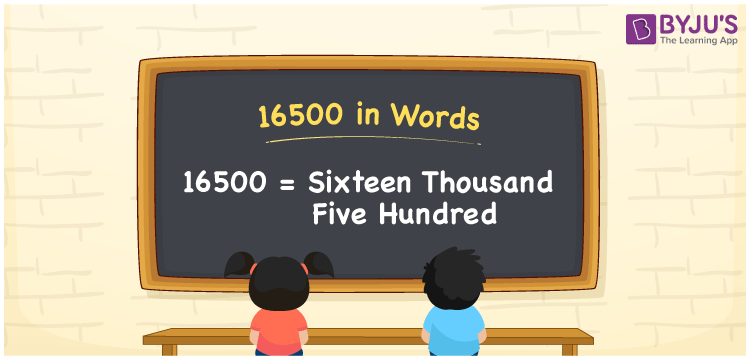# 16500 in words

16500 in words is written as Sixteen Thousand Five Hundred. 16500 represents the count or value in terms of numbers. The article on Counting Numbers can give you an idea about count or counting. The number 16500 is used in expressions that relate to money, distance, length and others. If expressed in terms of money, we can say that “ After all the deductions my earnings were Rupees Sixteen Thousand Five Hundred”.

 16500 in words Sixteen Thousand Five Hundred Sixteen Thousand Five Hundred in Numbers 16500

## 16500 in English Words## How to Write 16500 in Words?

We can convert 16500 to words using a place value chart. The number 16500 has 5 digits, so let’s make a chart that shows the place value up to 5 digits.

 Ten thousand Thousands Hundreds Tens Ones 1 6 5 0 0

Thus, we can write the expanded form as:

1 × Ten thousand + 6× Thousand + 5 × Hundred + 0 × Ten + 0 × One

= 1 × 10000 + 6 × 1000 + 5 × 100 + 0 × 10 + 0 × 1

= 16500

= Sixteen Thousand Five Hundred.

16500 is the natural number that is succeeded by 16499 and preceded by 16501.

16500 in words – Sixteen thousand five hundred.

Is 16500 an odd number? – No.

Is 16500 an even number? – Yes.

Is 16500 a perfect square number? – No.

Is 16500 a perfect cube number? – No.

Is 16500 a prime number? – No.

Is 16500 a composite number? – Yes.

## Solved Example

1. Write the number 16500 in expanded form

Solution: 1 x 10000 + 6 x 1000 + 5 x 100 + 0 x 10 + 0 x 1

Or Just 1 x 10000 + 6 x 1000 + 5 x 100

We can write 16500 = 10000 + 6000 + 500 + 0 + 0

= 1 x 10000 + 6 x 1000 + 5 x 100 + 0 x 10 + 0 x 1

## Frequently Asked Questions on 16500 in words

### How to write 16500 in words?

16500 in words is written as Sixteen Thousand Five Hundred.

### State True or False. 16500 is divisible by 4?

True. 16500 is divisible by 4.

### Is 16500 divisible by 10?

Yes. 16500 is divisible by 10. It is also divisible by 2, 4, 5, 10 and 100.